# Test: Electromagnetic Fields Theory- 2

## 20 Questions MCQ Test Mock Test Series for SSC JE Electrical Engineering | Test: Electromagnetic Fields Theory- 2

Description
Attempt Test: Electromagnetic Fields Theory- 2 | 20 questions in 12 minutes | Mock test for SSC preparation | Free important questions MCQ to study Mock Test Series for SSC JE Electrical Engineering for SSC Exam | Download free PDF with solutions
QUESTION: 1

### Two infinite parallel metal plates are charged with equal surface charge density of the same polarity. The electric field in the gap between the plates is

Solution:

Two infinite parallel metal plates are charged with an equal surface charge density of the same polarity. The equal surface charge densities are having the same charge either positive or negative. The same charge repels each other, hence the resultant electric field in the gap between the plates is zero.

QUESTION: 2

Solution:
QUESTION: 3

### An isolated sphere of radius 1 cm is kept in air. Its capacitance will be:

Solution:

The capacitance of an isolated sphere is,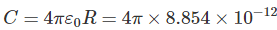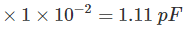QUESTION: 4

Which of the following is the unit of electric dipole moment

Solution:

The electric dipole moment is a measure of the separation of positive and negative electrical charges. It consists of two equal and opposite charges that are infinitely close together. Its magnitude is equal to the product of the charge and the distance between the charges and having direction from the negative to the positive charge along the line between the charges.

p = qd

The SI unit for electric dipole moment is coulomb-meter

QUESTION: 5

Which of the following is generally used to make permanent magnets?

Solution:

Materials that can be magnetized, which are also the ones that are strongly attracted to a magnet (ferromagnetic) are used to make a permanent magnet.

Cobalt steel contains about 35% of cobalt. It has a very good magnetic property that is why it is used for making permanent magnets.

QUESTION: 6

Copper, Silver, Diamond are examples of:

Solution:

Copper, Silver, Diamond are examples of diamagnetic materials.

Diamagnetic materials are slightly repelled by a magnetic field and the material does not retain the magnetic properties when the external field is removed.

QUESTION: 7

The dielectric strength of rubber is 30000 V/mm at a frequency of 50 Hz. What is the thickness of insulation required on an electrical conductor at 33 kV to sustain the breakdown?

Solution:

Given that,

Electric field (E) = 30000 V/mm

Breakdown voltage (V) = 33 kV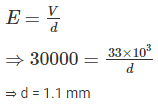QUESTION: 8

The λ is the flux linkage of a coil and I is the current through the coil then inductance of the coil is:

Solution:

The inductance of a coil is L = λ/I

Where, λ is flux linkage in weber-turns

I is current in amperes

QUESTION: 9

The current ratio (i2/i) for the circuit shown in fig. is:Solution: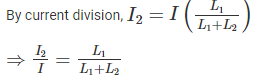QUESTION: 10

The direction of the magnetic lines of force around a current carrying conductor can be determined by:

Solution:

According to Right-hand thumb rule, imagine that you are holding a current carrying wire in your right hand so that the thumb points in the direction of the current, then the direction in which the fingers wrap the wire will represent the direction of magnetic lines of force.

QUESTION: 11

Permeability of the substance is the measure of:

Solution:

Permeability is the measure of the ability of a material to support the formation of a magnetic field within itself. It is the degree of magnetization that a material obtains in response to an applied magnetic field.

It is a constant of proportionality that exists between magnetic flux density and magnetic field intensity.

B = μH

The units of magnetic permeability are henry per meter

QUESTION: 12

The induced emf in a coil of 0.8 mH carrying 2A current and reversed in 0.4 second is:

Solution:

Given that,

Inductance of a coil (L) = 0.8 mH

Current (I) = 2 A

Change in current = 2 – (–2) = 4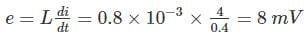QUESTION: 13

The differential coupling of two coils in series connection has self-inductance of 2 mH, 4 mH and a mutual inductance of 0.15 mH. The equivalent of the combination is:

Solution:

Given that, L1 = 2 mH, L2 = 4 mH, M = 0.15 mH

The equivalent of two coils in series connection for differential coupling is

Leq = L1 + L2 - 2M = 2 + 4 – 2 (0.15) = 5.7 mH

QUESTION: 14

The retentivity of a material useful for the construction of:

Solution:

This power of magnetism after the applied magnetism is removed is called retentivity of a substance.

Materials which are required to retain their magnetism will have high retentivity and as such are used to make permanent magnets.

QUESTION: 15

Higher the self-inductance of a coil

Solution:

Self-inductance of a coil is defined as the ratio of self-induced emf to the rate of change of current in the coil.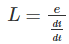Higher the self-inductance of a coil, longer the delay in establishing a steady current through it.

QUESTION: 16

The effect of an air gap in a magnetic circuit is to:

Solution:

The air gap in magnetic circuit means the magnetic resistance, i.e. reluctance to the magnetic flux density. Hence the air gap indicates the increase in reluctance.

QUESTION: 17

What is the MKS rationalized unit of magnetic pole strength quantity?

Solution:

Magnetic pole strength is a measure of the force exerted by one face of a magnet on a face of another magnet when both magnets are represented by equal and opposite poles.

Weber is the MKS rationalized unit of magnetic pole strength quantity.

QUESTION: 18

When the current in a coil is increased from 2 A to 4 A in 0.05 seconds, the e.m.f. induced in the coil is 8 V. The self-inductance of the coil is

Solution:

Given that,

Current (I) = Changed form 2 A to 4 A

Change in current = 2 A

Time (t) = 0.05

Emf (e) = 8 V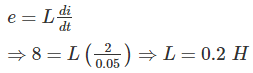QUESTION: 19

In the given circuit, inductance L1 and L2, if L1 = 2L2 and Leq is 0.7 H, are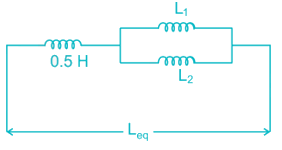Solution:

From the given circuit,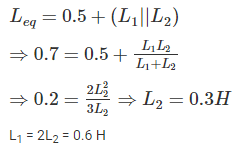QUESTION: 20

Soft magnetic materials have

Solution:

Soft magnetic materials are those materials that are easily magnetized and demagnetized.

Permeability is the measure of the ability of a material to support the formation of a magnetic field within itself. It is the degree of magnetization that a material obtains in response to an applied magnetic field. Hence it should be high for soft magnetic materials.

Coercive field or coercive force is a measure of the ability of a ferromagnetic material to withstand an external magnetic field without becoming demagnetized. Hence it should be low for soft magnetic materials.Use Code STAYHOME200 and get INR 200 additional OFF Use Coupon Code